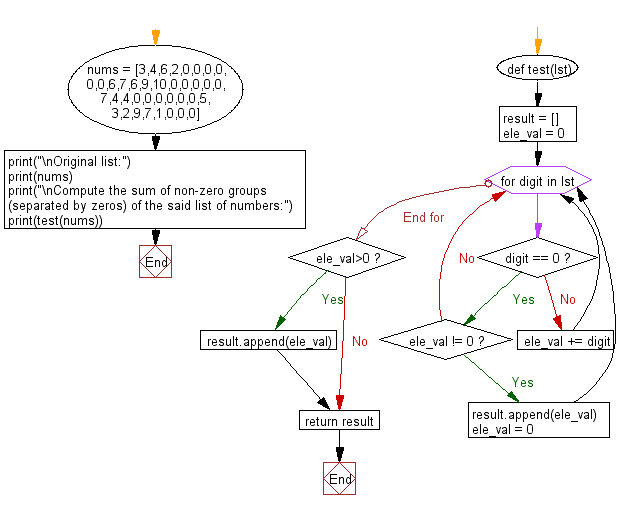﻿ Python: Compute the sum of non-zero groups (separated by zeros) of a given list of numbers - w3resource# Python: Compute the sum of non-zero groups (separated by zeros) of a given list of numbers

## Python List: Exercise - 210 with Solution

Write a Python program to compute the sum of non-zero groups (separated by zeros) of a given list of numbers.

Sample Solution:

Python Code:

``````def test(lst):
result = []
ele_val = 0
for digit in lst:
if digit == 0:
if ele_val != 0:
result.append(ele_val)
ele_val = 0
else:
ele_val += digit
if ele_val>0:
result.append(ele_val)
return result
nums = [3,4,6,2,0,0,0,0,0,0,6,7,6,9,10,0,0,0,0,0,7,4,4,0,0,0,0,0,0,5,3,2,9,7,1,0,0,0]
print("\nOriginal list:")
print(nums)
print("\nCompute the sum of non-zero groups (separated by zeros) of the said list of numbers:")
print(test(nums))
```
```

Sample Output:

```Original list:
[3, 4, 6, 2, 0, 0, 0, 0, 0, 0, 6, 7, 6, 9, 10, 0, 0, 0, 0, 0, 7, 4, 4, 0, 0, 0, 0, 0, 0, 5, 3, 2, 9, 7, 1, 0, 0, 0]

Compute the sum of non-zero groups (separated by zeros) of the said list of numbers:
[15, 38, 15, 27]
```

Flowchart:## Visualize Python code execution:

The following tool visualize what the computer is doing step-by-step as it executes the said program:

Python Code Editor:

Have another way to solve this solution? Contribute your code (and comments) through Disqus.

What is the difficulty level of this exercise?

Test your Python skills with w3resource's quiz

﻿

## Python: Tips of the Day

Floor Division:

When we speak of division we normally mean (/) float division operator, this will give a precise result in float format with decimals.

For a rounded integer result there is (//) floor division operator in Python. Floor division will only give integer results that are round numbers.

```print(1000 // 300)
print(1000 / 300)```

Output:

```3
3.3333333333333335```